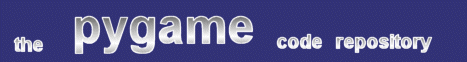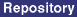### Title: Meltdown

Author: Sebastian "WhiteTiger" John (TheWhiteTiger at gmx.net)
Submission date: September 29, 2002

Description: A port of the 'Melt' effect.pygame version required:
SDL version required:
Python version required:

Comments: This script ports the well-known 'melt' effect, familiar to users of the 'xmelt' program, and to players of Doom.

```
"""
Name:		Meltdown
Author:		Sebastian "WhiteTiger" John
Email:		TheWhiteTiger@gmx.net

This is public domain code.

Meltdown -- 'mess up the screen' (cite by David Lemke). This effect takes a
surface, moves blocks from the top downwards and fills the place where the
block has been with black until the whole surface is black. If you want to
use it in your own code, have a look at the definition of 'main()'.

This is a port of the linux/Xlib program 'xmelt' by Dave Lemke. I did almost
nothing more than porting the C/Xlib stuff to python/pygame. Unfortunately
my port is much slower than the original code... That means that it doesn't
need any pygame.time stuff at all ;)
"""

import random
import pygame
from pygame.locals import *

def myrandom(n):
# another layer of overhead...
return random.randrange(n)

class Meltdown:
def __init__(self, surface, min_size=10, max_size=100, min_dist=10,

self.surf = surface

self.WIDTH, self.HEIGHT = surface.get_size()

self.MIN_SIZE = min_size
self.MAX_SIZE = max_size

self.MIN_DIST = min_dist

self.MIN_WIDTH = min_width

self.MAX_HEIGHT = self.HEIGHT - self.MIN_SIZE
self.FINISHED = finished

self.heights = []
while len(self.heights) < self.WIDTH: self.heights.append(0)

self.finished = 0

# this procedure is only for historical reasons
# it is now inlined because of speed
#def calc_xloc(self, width):
#    # original version
#    #xloc = rand(self.WIDTH + self.MIN_WIDTH) - self.MIN_WIDTH
#    #xloc = rand(self.WIDTH) - self.MIN_WIDTH
#    #xloc = rand(self.WIDTH) - 2*self.MIN_WIDTH
#    #xloc = rand(self.WIDTH)
#    # this one seems to look best:
#    xloc = rand(self.WIDTH + self.MIN_WIDTH) - 2*self.MIN_WIDTH
#    return max(min((xloc + width), (self.WIDTH - width)), 0)

def step(self):
# redefinitions to speed things up a little bit
WIDTH = self.WIDTH
MIN_WIDTH = self.MIN_WIDTH
HEIGHT = self.HEIGHT
MAX_HEIGHT = self.MAX_HEIGHT

rand = myrandom
heights = self.heights
surf = self.surf

_range = range
_min = min
_max = max

# precalculations
width = rand(MIN_WIDTH) + self.WIDTH_ADD

#xloc = self.calc_xloc(width)
xloc = rand(WIDTH + MIN_WIDTH) - 2*MIN_WIDTH
xloc = _max(_min((xloc + width), (WIDTH - width)), 0)

yloc = HEIGHT
for i in _range(xloc, (xloc + width)):
yloc = _min(yloc, heights[i])
if yloc == HEIGHT:
return surf, []

# calculate block size
dist = rand(yloc/10 + self.MIN_DIST)
size = rand(_max(yloc + self.MIN_SIZE, self.MAX_SIZE))

# define rects for blitting
destpos = (xloc, yloc + dist)
destrect = (xloc, yloc, width, dist)
sourcerect = (xloc, yloc, width, size)

# the visible bit
surf.blit(surf, destpos, sourcerect)
surf.fill((0, 0, 0), destrect)

# postcalculations
yloc += dist
for i in _range(xloc, (xloc + width)):
if heights[i] < MAX_HEIGHT and yloc >= MAX_HEIGHT:
self.finished += 1
heights[i] = _max(heights[i], yloc)

if self.finished >= (WIDTH - self.FINISHED):
return None, []

# everything is fine, return the new surface
return surf, [sourcerect, destrect]

def main():
# resolution
#RES = (640, 480)
RES = (320, 240)
#RES = (160, 120)

# pygame initialization
pygame.init()
screen = pygame.display.set_mode(RES)

# load and convert an image
image = pygame.transform.scale(image, RES)

# blit the surface to the screen
screen.blit(image, (0,0))
pygame.display.flip()

# instantiate the Meltdown class
meltdown = Meltdown(image)

# first step
surface, dirty = meltdown.step()

# main loop
while surface:
# event stuff
for ev in pygame.event.get():
if ev.type in (QUIT, KEYDOWN, MOUSEBUTTONDOWN):
return

# redraw screen
screen.blit(surface, (0,0))
pygame.display.update(dirty)

# next step
surface, dirty = meltdown.step()

if __name__ == "__main__":
main()

```

 Feedback